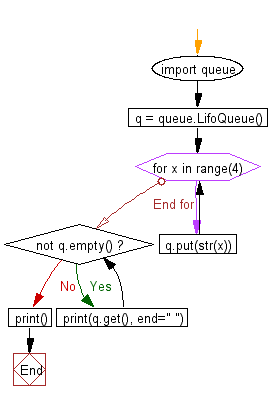﻿ Python heap queue algorithm: Create a LIFO queue - w3resource

# Python: Create a LIFO queue

## Python heap queue algorithm: Exercise-29 with Solution

Write a Python program to create a LIFO queue.

Sample Solution:

Python Code:

``````import queue
q = queue.LifoQueue()
#insert items at the head of the queue
for x in range(4):
q.put(str(x))
#remove items from the head of the queue
while not q.empty():
print(q.get(), end=" ")
print()
```
```

Sample Output:

```3 2 1 0
```

Flowchart:Python Code Editor:

Have another way to solve this solution? Contribute your code (and comments) through Disqus.

What is the difficulty level of this exercise?

Test your Programming skills with w3resource's quiz.

﻿